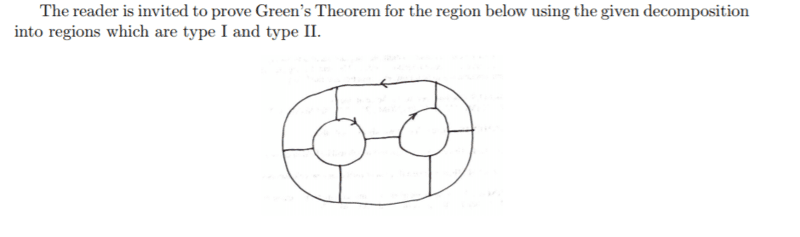# Question about type 1 and type 2 regions

• Clara Chung
In summary, the given decomposition has regions that are both type 1 and type 2 at the same time. This is because the functions bounding the curves are not continuous, but rather two piece or piecewise continuous functions. For example, the upper right hand region has an upper curve that is the corner curve and a lower curve that is made up of an arc of a circle and a horizontal line. It would require two integrals, either as a dx or dy integral, to cover this region. Similarly, the lower curve in this region is the corner curve and the upper curve is made up of a vertical line segment and an arc of the inner circle, requiring two integrals as well. Despite the sharp corners, each segment is still

## Homework Statement## The Attempt at a Solution

How is the regions in the given decomposition both type 1 and type 2 regions at the same time? Take the region at the upper right hand side as an example. It can't be neither type 1 and type 2 regions because the functions that are bounding the curve are not continuous. e.g. If I take the rightmost point as x=b, leftmost line as x=a, the lower function bounding y is not continuous.
•